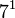# Groups of order 112

## Contents

See pages on algebraic structures of order 112| See pages on groups of a particular order

## Statistics at a glance

The number 112 has prime factors 2 and 7. The prime factorization is as follows:$\! 112 = 2^4 \cdot 7^1 = 16 \cdot 7$

There are only two prime factors of this number. Order has only two prime factors implies solvable (by Burnside's$p^aq^b$-theorem) and hence all groups of this order are solvable groups (specifically, finite solvable groups). Another way of putting this is that the order is a solvability-forcing number. In particular, there is no simple non-abelian group of this order.

Quantity Value Explanation
Total number of groups up to isomorphism 43
Number of abelian groups up to isomorphism 5 (number of abelian groups of order$2^4$)$\times$ (number of abelian groups of order$7^1$) = (number of unordered integer partitions of 4)$\times$ (number of unordered integer partitions of 1) =$5 \times 1 = 5$.
Number of nilpotent groups up to isomorphism 14 (number of groups of order 16)$\times$ (number of groups of order 7) =$14 \times 1 = 14$
Number of supersolvable groups up to isomorphism 42
Number of solvable groups up to isomorphism 43 There are only two prime factors of this number. Order has only two prime factors implies solvable (by Burnside's$p^aq^b$-theorem) and hence all groups of this order are solvable groups (specifically, finite solvable groups). Another way of putting this is that the order is a solvability-forcing number. In particular, there is no simple non-abelian group of this order.

## GAP implementation

The order 112 is part of GAP's SmallGroup library. Hence, any group of order 112 can be constructed using the SmallGroup function by specifying its group ID. Also, IdGroup is available, so the group ID of any group of this order can be queried.

Further, the collection of all groups of order 112 can be accessed as a list using GAP's AllSmallGroups function.

Here is GAP's summary information about how it stores groups of this order, accessed using GAP's SmallGroupsInformation function:

gap> SmallGroupsInformation(112);

There are 43 groups of order 112.
They are sorted by their Frattini factors.
1 has Frattini factor [ 14, 1 ].
2 has Frattini factor [ 14, 2 ].
3 - 18 have Frattini factor [ 28, 3 ].
19 - 26 have Frattini factor [ 28, 4 ].
27 - 36 have Frattini factor [ 56, 12 ].
37 - 40 have Frattini factor [ 56, 13 ].
41 - 43 have trivial Frattini subgroup.

For the selection functions the values of the following attributes
are precomputed and stored:
IsAbelian, IsNilpotentGroup, IsSupersolvableGroup, IsSolvableGroup,
LGLength, FrattinifactorSize and FrattinifactorId.

This size belongs to layer 2 of the SmallGroups library.
IdSmallGroup is available for this size.